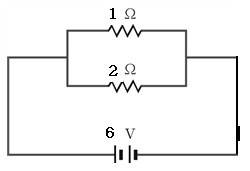# Which bulb is really more collision of electrons inparallel connectionWhich bulb is really more collisions of electrons inparallel connection?

The intensity of bulb's light is depend on the power of electricity(IV), so the upper blub has more number of collisons between electrons and atoms.

but I think actually we consider about the numer of collisions
the upper bulb flows 6A = 6*6.25*10^18 electrons, the resistor length is 1
the lower bulb flows 3A = 3*6.25*10^18 electrons, the resistor length is 2
so totally the number of collisions is same.
so I think the intensity of two bulbs is same.

How can I solve this problem?

#### Attachments

UltrafastPED
Gold Member
Consider the power being expended in each branch: P=VI.

Because your bulbs are in parallel the voltage is the same for each bulb: V=6.

Now which bulb has more power? What is the power being used for? Which bulb is brighter?

Consider the power being expended in each branch: P=VI.

Because your bulbs are in parallel the voltage is the same for each bulb: V=6.

Now which bulb has more power? What is the power being used for? Which bulb is brighter?

But I want to solve the question by the other concept that the electron's collision number with atoms of resistor.

meBigGuy
Gold Member
First, explain electron collisions, and explain their significance. Also, explain, since you know the 1ohm resistor is equivilent to two 2 ohm resistors in parallel, what collisions have to do with anything.

UltrafastPED
Gold Member
But I want to solve the question by the other concept that the electron's collision number with atoms of resistor.

If you start with the Drude model of the electron gas and a bit of statistical mechanics you can derive an intuitive understanding of resistors, but this is the long way around the barn.

You already have the result, which is Ohm's law. Here you need to learn how to apply it to the problem at hand.

Once you have the correct answer by means of Ohm's law you can go back and see if you are able to get there from the Drude model. Of course the Drude model is incorrect in detail, but it does give you a feel for things. But the quantum model can wait until you take a condensed matter course plus the quantum version of statistical mechanics.

If you start with the Drude model of the electron gas and a bit of statistical mechanics you can derive an intuitive understanding of resistors, but this is the long way around the barn.

You already have the result, which is Ohm's law. Here you need to learn how to apply it to the problem at hand.

Once you have the correct answer by means of Ohm's law you can go back and see if you are able to get there from the Drude model. Of course the Drude model is incorrect in detail, but it does give you a feel for things. But the quantum model can wait until you take a condensed matter course plus the quantum version of statistical mechanics.

So can I say that the point of my misunderstanding is from the drude model?
It is incorrect theory for solving this problem.
And I have to approach that problem by ohm's law.

The intensity of bulb's light is only depend on How much electric power(P=IV) is.
And Don't try to solve this problem by number of collisons.

Is it right my conclusion?

UltrafastPED
Gold Member
Physical Origins of Ohm's Law

Ohm's law already includes the collisions (or better, the scattering), so for ordinary problems it gives the correct answer.

Attached are some notes on the physical origins of Ohm's Law.

Last edited:
•1 person
meBigGuy
Gold Member
Nice paper: Always learning :)
It seems that (in the simple cases) the resistance is based more on the mean free path than on the number of collisions, which has a nice intuitive feel.